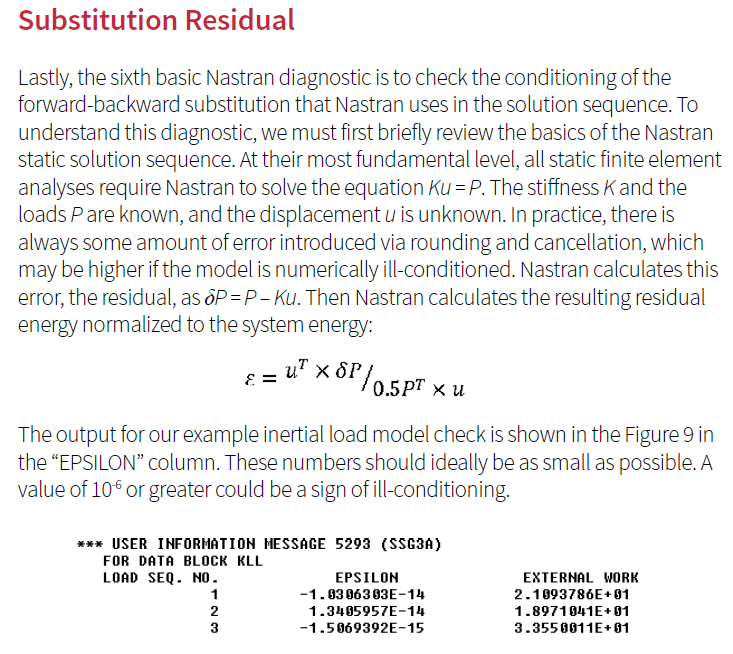peteroznewman
Subscriber
nHere is the definition of Nastran's Epsilon check, also known as...nTo calculate this in ANSYS, you have to do the matrix math to calculate deltaP, then divide by the system energy to normalize it to get epsilon. I don't know how to do that.n